#### Structure and Bonding in semiconductors, Physics tutorial

Introduction:

In a semiconductor, the valence band is nearly completely filled whereas the conduction band is empty. Thermal excitation or (energy) absorption methods might cause some of the electrons to cross the band gap, making it identical to semimetals. The semiconductors tend to be bonded tetrahedral and covalently, however binary semiconductors might encompass polar and also covalent character.

Definition:

Semiconductors are basically the electronic conductors having electrical resistivity values usually in the range of 10-2 to 109 ohm-cm at room temperature, intermediate between the good conductors (10-6 ohm-cm) and insulators (1014 - 1022 ohm-cm).

Crystal structure and bonding:

The semiconductors comprise a huge number of substances of broadly different chemical and physical properties. Such materials are grouped into various classes of similar behavior, the categorization being based on the position in the periodic table of the elements.

The best-recognized class is the Group IV semiconductors - C (diamond), Si, Ge, - all of which lie in the fourth column of the periodic table. They have been studied intensively, specifically Si and Ge that have found numerous applications in the electronic devices. The elemental semiconductors all crystallize in the diamond structure. The diamond structure consists of an fcc lattice having a basis comprised of two similar atoms, and is such that each and every atom is surrounded via four neighboring atoms, making a regular tetrahedron. Group IV semiconductors are covalent crystals, that is, the atoms are held together through covalent bonds. Such bonds comprise of two electrons of opposite spins distributed all along the line joining the two atoms. The covalent electrons making the bonds are hybrid sp3 atomic orbital.

The other significant group of semiconductors is the Group III-V compounds, so named as each and every contains two elements, one from the third and the other from the fifth column of the periodic table. The best-recognized members of this group are GaAs and InSb (that is, indium antimonite); however the list as well includes compounds like GaP, InAs, GaSb and lots of others.

Such substances crystallize in the zinc blend structure that is similar as the diamond structure; apart from that the two atoms making the basis of the lattice are now different. Therefore, in GaAs, the base of the fcc lattice comprises of two atoms, Ga and As. Due to this structure, each and every atom is surrounded via four others of the opposite type, and such latter atoms form a regular tetrahedron, just as in the structure of diamond.

The bonding in the III-V compounds is as well mainly covalent. The eight electrons needed for the four tetrahedral covalent bonds are supplied via the two kinds of atoms, the trivalent atom contributing its three valence electrons, and the pentavalent atom five electrons. The bonding in this group is not completely covalent. As the two elements in the compound are dissimilar, the distribution of the electrons all along the bond is not symmetric, however is displaced toward one of the atoms. As an outcome, one of the atoms achieves a total electric charge. Such a bond is termed as heteropolar, in dissimilarity to the purely covalent bond in the elemental semiconductors that is termed as homopolar.

The distribution of electrons in the bond is relocated toward the atom of higher electronegativity. In GaAs, for example, the As atom consists of a higher electro-negativity than the Ga, and as a result the As atom obtains a total negative charge, whose value is - 0.46e per atom (that is, a typical value in Group III-V compounds). The Ga atom correspondingly obtains a total positive charge of 0.46e. Charge transfer leads to the ionic contribution to the bonding in Group III-V compounds. Their bonding is thus in reality a mixture of covalent and ionic components, however covalent ones predominate in most of such substances.

Bonding structure:

Semiconductor is a solid in which the maximum occupied energy band, the valence band, is wholly full at T = 0°K, however in which the gap above this band is as well small, in such a way that electrons might be excited thermally at room temperature from the valence band to the next-higher band that is termed as the conduction band. Usually speaking, the number of excited electrons is appreciable (at room temperature) if the energy gap EF is less than 2 eV. The substance might then be categorized as a semiconductor. If the gap is bigger, the number of electrons is negligible, and the substance is an insulator. If electrons are excited across the gap, then the bottom of conduction band (CB) is populated via electrons, and the top of the valence band (VB) by holes. As an outcome, both the bands are now just partially full, and would carry a current whenever an electric field was applied. The conductivity of the semiconductor is small compared by the conductivities of metals of the small number of electrons and holes comprised, however this conductivity is however adequately large for practical purposes.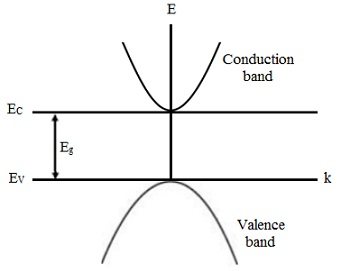The simplest band structure of a semiconductor is represented in the figure above. As we are interested only in the area that lies close to the band gap, where electrons and holes lie, we can avoid a more complicated variation of the energy bands far away from the gap. The energy of the CB consists of the form.

The energy of the Conduction band has the form:

EC(k) = EC + [(h/2π)2k2]/2me

Here 'k' is the wave vector and me the effective mass of the electron. The energy Eg symbolizes the energy gap. The zero-energy level is selected to lie at the top of the valence band. The energy of the valence band might be represented as:

Ev(k) = Ev - [(h/2π)2k2]/2mh

Here mh is the effective mass of the hole that is positive. (Due to the inverted shape of the Valence band, the mass of an electron at the top of the Valence band is negative; however the mass of a hole is positive.)

In this simple picture of the semiconductor, the primary band-structure parameters are therefore the electron and hole masses me and mh, and the band gap Eg. The table illustrated below gives such parameters for different semiconductors. Note that the masses vary considerably from the free-electron mass. In most of the cases they are much smaller than the free-electron mass. The energy gaps range from 0.18 eV in InSb to 3.7 eV in ZnS. The table as well represents that the wider the gap, the greater the mass of the electron.

Table: Band Structure parameters of Semiconductors

 Group Crystal Eg(eV) me/m mh/m IV C 5.3 IV Si 1.1 0.19 0.16 IV Ge 0.7 0.08 0.04 III-V GaAs 1.4 0.07 0.09 III-V GaP 2.3 0.12 0.50 III-V InSb 0.2 0.01 0.18 II-VI ZnS 3.6 0.40 5.41 II-VI ZnSe 2.7 0.1 0.6 II-VI CdSe 1.7 0.13 0.45

The energy gap for the semiconductor differs by temperature; however the variation is generally slight. That a variation by temperature must exist at all can be appreciated from the fact that the crystal, if it is heated, undergoes a volume expansion and therefore a change in its lattice constant. This, in turn, influences the band structure that is a sensitive function of the lattice constant.

The band structure in the figure above is the simplest possible structure. Band structures of real semiconductors are rather more complex, as we shall observe however for the present the simple structure will suffice for our aims.

Intrinsic Semiconductors:

In the field of semiconductors, electrons and holes are generally termed to as the free carriers, or simply carriers, as it is such particles that are responsible for carrying the electric current. The number of carriers is a significant property of a semiconductor, as this finds out its electrical conductivity. Intrinsic semiconductors are the semiconductors in which the number of carries and the conductivity is not affected via impurities. Intrinsic conductivity is typical at relatively high temperatures in highly purified specimens.

In order to find out the number of carriers, we require some of the fundamental results of the statistical mechanics. The most significant outcome in this regard is the Fermi-Dirac (FD) distribution function:

f(E) = 1/[e(E-μ)/kBT + 1]

This above function gives the probability that an energy level 'E' is occupied through an electron if the system is at temperature 'T'.

The function is plotted versus E in the figure shown below. Here we observe that, as the temperature increases, the unoccupied region beneath the Fermi level EF becomes longer that means that the occupation of high energy states increases as the temperature is increased, a conclusion that is most plausible, as increasing the temperature increases the overall energy of the system.We will observe later that the Fermi level in intrinsic semiconductors lies close to the middle of the band gap. Thus we can represent the distribution function and the conduction and valence bands of the semiconductor as represented in the figure given below.First we compute the concentration of electrons in the CB. The number of states in the energy range (E, E + dE) is equivalent to De(E) dE, here De(E) is the density of electron states. As each of these states consists of an occupation probability f(E), the number of electrons in reality found in this energy range is equivalent to f(E)De(E)dE. The concentration of electrons all through the Conduction band is therefore given via the integral over the conduction band.

n = EC fe(E) De (E) dE

Here, Ec is the bottom of the conduction band, as illustrated in the figure above.

The band gap in semiconductors is of the order of 1eV that is much bigger than kT. Thus (E-µ) >> kBT and we can ignore the unity term in the denominator of the distribution function so that,

fe(E) ≈ e-(E-μ)/kBT

The density of states for the conduction band is represented by: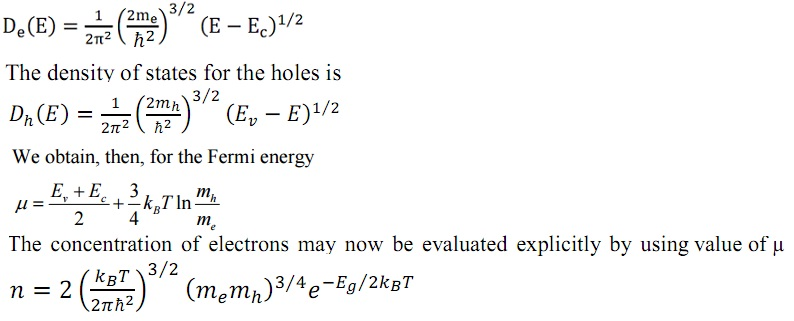Here, Eg = EC - Ev is the band gap. The significant characteristic of this expression is that 'n' rises very rapidly - exponentially - with temperature, specifically by virtue of the exponential factor. Therefore as temperature is increased, a vastly greater number of electrons are excited across the gap. Our explanation of carrier concentration in this part is mainly based on the premise of a pure semiconductor. If the substance is impure, additional electrons or holes are given by the impurities. In that condition, the concentrations of electrons and holes might no longer be equivalent, and the amount of each and every depends on the concentration and kind of impurity present. If the substance is sufficiently pure so that the concentrations of electrons and holes are equivalent, we speak of an intrinsic semiconductor. That is, the concentrations are determined through the intrinsic properties of the semiconductor itself. On the other hand, if a substance includes a large number of impurities that supply most of the carriers, it is termed to as the extrinsic semiconductor.

Impurity states:

A pure semiconductor consists of equivalent numbers of both kinds of carriers, electrons and holes. In most of the applications, though, one requires specimens that have one kind of carrier only, and none of the other. By doping the semiconductor by suitable impurities, one can get samples that have either electrons only or holes only.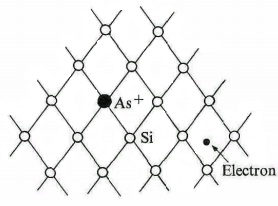Consider, for example, a specimen of Si which has been doped via As. The As atoms (that is, the impurities) occupy some of the lattice sites formerly occupied via the Si host atoms. The distribution of the impurities is random all through the lattice. However their presence influences the solid in one very significant respect. The As atom consists of valence 5 whereas Si consists of valence 4. Of the five electrons of As, four participate in the tetrahedral bond of Si, as represented in the figure shown below. The fifth electron can't enter the bond that is now saturated, and therefore this electron detaches from the impurity and is free to migrate via the crystal as a conduction electron, that is, the electron enters the CB. The impurity is now in reality a positive ion, As+ (as it consists of lost one of its electrons), and therefore it tends to capture the free electron, however we shall show shortly that the attraction force is extremely weak, and not adequate to capture the electron in most conditions.

The total result is that the As impurities contribute electrons to the CB of the semiconductors, and for this reason such impurities are termed as donors. It will be noted that the electrons have been created devoid of the generation of holes.

If an electron is captured via an ionized donor, it orbits around the donor much similar to the situation in hydrogen. We can compute the binding energy by employing the familiar Bohr model. Though, we must take into account the fact that the coulomb interaction here is weakened via the screening due to the presence of the semiconductor crystal that serves as a medium in which both the donor and ion reside. Therefore the coulomb potential is now represented by:

V(r) = - e2/εr

Here 'ε' is the reduced dielectric constant of the medium. The dielectric constant ε = 11.7 in Si, for illustration, represents a substantial decrease in the interaction force. It is this screening that is responsible for the small binding energy of the electron at the donor site.

By employing this potential in the Bohr model, we determine the binding energy, corresponding to the ground state of the donor, to be:

Ed = - e4me/2ε2(h/2π)2

It will be noted that the effective mass 'm' has been employed instead of the free mass 'm'. The binding energy of the hydrogen factor 1/ε2, and as well through the mass factor me/m that is generally smaller than unity. By employing the typical values ε ~ 10 and me/m ~ 0 .001 eV. This is certainly the order of the observed values.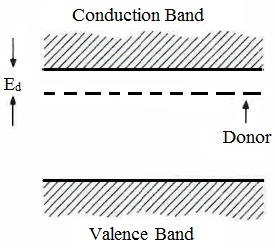The donor level lies in the energy gap, very slightly beneath the conduction band, as illustrated in the figure shown above. As the level is so close to the Conduction band, nearly all the donors are ionized at room temperature, their electrons having been excited to the Conduction band.

This is instructive to compute the Bohr radius of the donor electron.Straightforward adaptation of the Bohr result leads to:

rd = ε (m/me) ao

Here a0 is the Bohr radius, equivalent to 0.53 Å. The radius of the orbit is therefore much bigger than a0, by a factor of 100, if we employ the prior values for ε and me. A typical radius is therefore of the order of 50 Å. As this is much bigger than the interatomic spacing, the orbit of the electron encloses a great many host atoms, and our picture of the lattice acting as the continuous, polarizable dielectric is therefore a plausible one.

As the donors are nearly all ionized, the concentration of electrons is nearly equivalent to that of the donors. Typical concentrations are around 1015 cm3. However at times much higher concentrations are obtained through heavy doping of the sample, for illustration, 1018 cm3 or even more.

Acceptors:

The appropriate choice of impurity might generate holes rather than electrons. Assume that the Si crystal is doped by Ga impurity atoms. The Ga impurity resides at a site formerly occupied through Si atom, however as Ga is trivalent, one of the electron bonds remains vacant (figure shown below). This vacancy might be filled via an electron moving in from another bond, resultant in a vacancy (or hole) at this latter bond. The hole is then free to migrate all through the crystal. In this way, by introducing a huge number of trivalent impurities, one makes an appreciable concentration of holes that lack electrons.

The trivalent impurity is termed as an acceptor, as it accepts an electron to complete its tetrahedral bond. The acceptor is negatively charged, by virtue of the additional electron it has entrapped. As the resultant hole consists of a positive charge, it is attracted by the acceptor. We can assess the binding energy of the hole at the acceptor in the same way followed above in the case of the donor. Again this energy is extremely small, of the order of 0.01 eV. Therefore essentially all the acceptors are ionized at room temperature.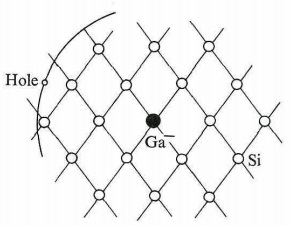The acceptor level lies in the energy gap, somewhat above the edge of the Valence band, as illustrated in the figure given above. This level corresponds to the hole being captured via the acceptor. If an acceptor is ionized (that is, an electron excited from the top of the Valence band to fill this hole), the hole falls to the top of the VB, and is now a free carrier. Therefore the ionization method, indicated via upward transition of the electron on the energy scale, might be illustrated by a downward transition of the hole on this scale.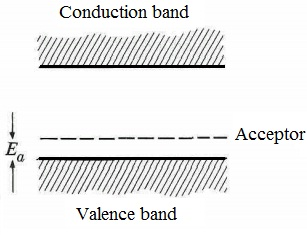Tutorsglobe: A way to secure high grade in your curriculum (Online Tutoring)